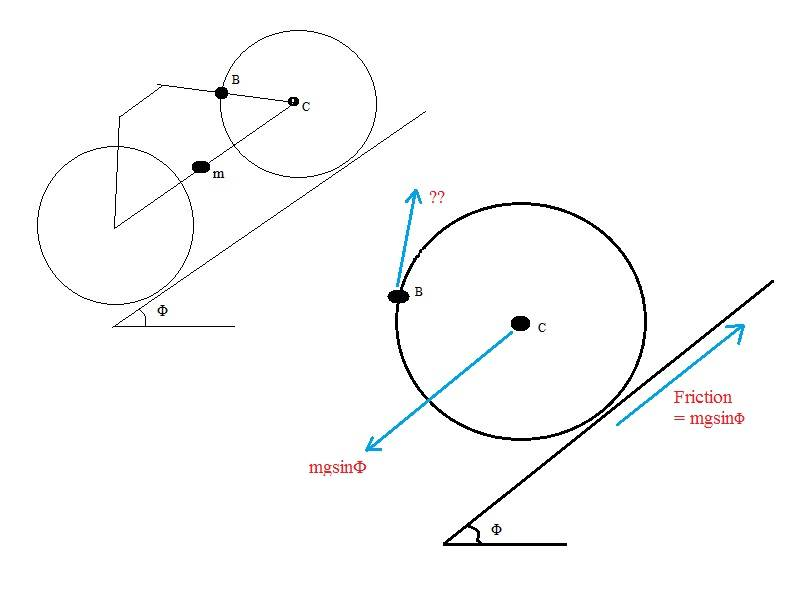# Balancing forces on a bicycle wheel

Hi, (this is not a homework question)

If I have a bicycle with COM, M (in line with the center of the wheels, C) on an incline, angle Φ, and the bicycle is NOT moving at all because the brake is on at B.

I am trying to put in the forces acting on the wheel. I figure that the weight of the bicycle is probably acting through the center of the wheel because the COM is at the same height (would this change if the COM was in a different place?). And because the wheel is not moving laterally I figure the friction force is opposing the weight, but acting at ground level.

The brake is preventing the wheel from turning CCW, and so I think the ?? force from the brake needs to also be mgsinΦ in a CW direction. But the problem is that if I put the brake/?? force in, the static/overall forces on the wheel won't balance and the wheel should move off in the direction of the ?? force.

Help!?
thanksK^2
I figure that the weight of the bicycle is probably acting through the center of the wheel because the COM is at the same height (would this change if the COM was in a different place?). And because the wheel is not moving laterally I figure the friction force is opposing the weight, but acting at ground level.
First of all, force from the frame is acting on center, right. That's property of the axis, so it will not change even if CoM is higher/lower.

You are also correct that the friction will be equal to weight's projection along the incline. No problem there.

What you are forgetting about is Newton's 3rd Law for the brake. If brake is applying force on wheel, wheel is applying force back on the brake. That force would accelerate the bike backwards if it weren't attached to the wheel. So rather than just bike's weight, front wheel experiences bike's weight plus the reaction force of the brake through it's center.

Now you can balance the tangential forces. Keep in mind that you also have normal forces on the wheel, and that normal force on the two wheels together adds up to component of weight perpendicular to the incline.

What you are forgetting about is Newton's 3rd Law for the brake. If brake is applying force on wheel, wheel is applying force back on the brake.

But the whole thing is stationary, so Newton's 3rd applies to everything! So that doesn't help me: The wheel is pushing on the ground equally and opposite to the Friction force pushing on the wheel, and the wheel is pulling on the bicycle mass equally and opposite to the bicycle mass pulling on the wheel.

What I am trying to do is find the forces acting on the wheel.
The wheel is not moving laterally, so the friction force must be opposed equally by the bicycle weight.
The wheel is not rotating, so the torque due to the friction and the weight force must be opposed equally by the torque from the brake? How do you work these out?

thanks,
p

HallsofIvy